## 3. 4. 1 Conventional Method

Again, the Laplace operator is considered for the specification of a finite difference scheme. A typical formula for a finite difference scheme may have the following shape: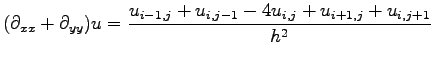(3.51)

From this formulation the following implicit assumptions are taken: Firstly, a quantity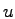is defined on a grid which is located by two independent indices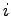and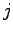indicating discrete values on the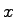as well as the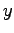axis. Furthermore, finite difference schemes are restricted to grids, namely to topological structures in which each vertex is implicitly assigned neighboring vertices by the structure of the grid.

If the distance between two neighboring vertices, here denoted as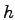is equal for all vertices, independently from the direction, the formulae can be written in a very simple manner. Otherwise, the distance between the single vertices has to be calculated separately either by using the length of the edges or alternatively by explicitly using the vertex coordinates.

Michael 2008-01-16